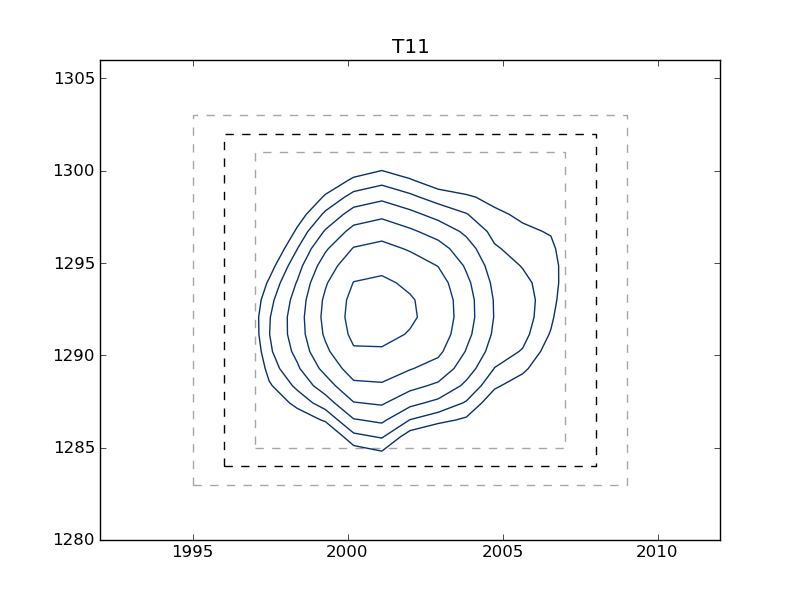# plotting example: plot_2d_boxes

This example shows how to use nmrglue and matplotlib to create figures for examining data or publication. In this example the box limits used in integration example: integrate_2d are graphically examined. A contour plot of each peak is plotted with the box limits indicated by the dark dashed line. To check peak assignments see plotting example: plot_2d_assignments.

```#! /usr/bin/env python
# Create a contour plots of each peak defined in limits.in file

import nmrglue as ng
import numpy as np
import matplotlib.pyplot as plt
import matplotlib.cm

# plot parameters
cmap = matplotlib.cm.Blues_r    # contour map (colors to use for contours)
contour_start = 30000           # contour level start value
contour_num = 20                # number of contour levels
contour_factor = 1.20           # scaling factor between contour levels

# calculate contour levels
cl = contour_start * contour_factor ** np.arange(contour_num)

# read in the data from a NMRPipe file

# read in the integration limits
peak_list = np.recfromtxt("limits.in")

# loop over the peaks
for name, x0, y0, x1, y1 in peak_list:

if x0 > x1:
x0, x1 = x1, x0
if y0 > y1:
y0, y1 = y1, y0

# slice the data around the peak

# create the figure
fig = plt.figure()

# plot the contours
print "Plotting:", name
ax.contour(slice, cl, cmap=cmap, extent=etup)

# draw a box around the peak
ax.plot([x0, x1, x1, x0, x0], [y0, y0, y1, y1, y0], 'k--')

# draw light boxes at +/- one point
ax.plot([x0 - 1, x1 + 1, x1 + 1, x0 - 1, x0 - 1],
[y0 - 1, y0 - 1, y1 + 1, y1 + 1, y0 - 1], 'k--', alpha=0.35)
ax.plot([x0 + 1, x1 - 1, x1 - 1, x0 + 1, x0 + 1],
[y0 + 1, y0 + 1, y1 - 1, y1 - 1, y0 + 1], 'k--',alpha=0.35)

# set the title
ax.set_title(name)

# save the figure
fig.savefig(name + ".png")
del(fig)
```
```#Peak   X0      Y0      X1      Y1
# Peak defines 15N resonance in 2D NCO spectra.
# Limits are in term of points from 0 to length-1.
# These can determined from nmrDraw by subtracting 1 from the X and Y
# values reported.
#Peak   X0      Y0      X1      Y1
T49     1992    1334    2003    1316
T11     1996    1302    2008    1284
# comments can appear anywhere in this file just start the line with #
G14     2032    1314    2044    1293
E15     2077    1025    2087    1004
W43     2008    952     2019    933
```

Sample Figure